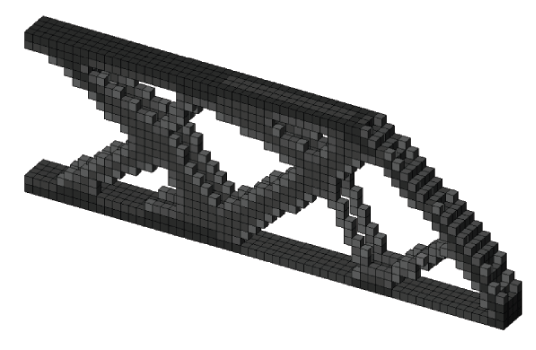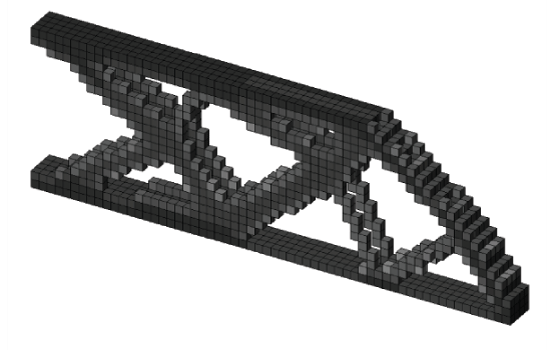# [Filters] Sensitivity filter - Top3d

In the topology optimization, filters are introduced to avoid numerical instabilities. Different filtering techniques may result different discreteness of the final solutions, and sometimes may even contribute to different topologies. In this series of tutorial, you will learn how to implement sensitivity filter and gray scale filter in the top3d program.

In the topology optimization, filters are introduced to avoid numerical instabilities. Different filtering techniques may result different discreteness of the final solutions, and sometimes may even contribute to different topologies. In addition to density filter, in the literatures there are bunch of different filtering schemes. For example, sensitivity filter, morphology based black and white filters, filtering technique using Matlab built-in function conv2, filtering based on Helmholtz type differential equations, Heaviside filter, and gray scale filter. All the filters pursue a simple goal to achieve black-and-white structures. Two of them are chosen, which stand for classic and better performance, as well as easy implementation.

Simgund introduced the sensitivity filter. The working principle is to replace the real sensitivities by the filtered sensitivities. In addition, the sensitivity filter is implemented in the 99-line code as the default filtering scheme. It modifies the element sensitivity during every iteration by the following

where $\gamma$ $(=10^{-3})$ is a small number in order to avoid division by zero.

The implementation of the sensitivity filter can be achieved by adding and changing a few lines.

### Step.1: Adding One Input Variable

Change line 2 by adding one input variable $\texttt{ft}$ ($\texttt{ft} = 1$ for density filter, $\texttt{ft} = 2$ for sensitivity filter)

function top3dft(nelx,nely,nelz,volfrac,penal,rmin,ft)


### Step.2: Adding the Sensitivity Filter

In order to add the sensitivity filter to the program, we need to change lines 79-80:

if ft == 1
dc(:) = H*(dc(:)./Hs);
dv(:) = H*(dv(:)./Hs);
elseif ft == 2
dc(:) = H*(x(:).*dc(:))./Hs./max(1e-3,x(:));


### Step.3: Changing OC subroutine

Changing the design variable update strategy (line 86) in the optimal search procedure

if ft == 1
xPhys(:) = (H*xnew(:))./Hs;
elseif ft == 2
xPhys = xnew;


### Step.4: Results

The default cantilever beam program solved using sensitivity filter is shown below (left) compared with those using density filter (right)